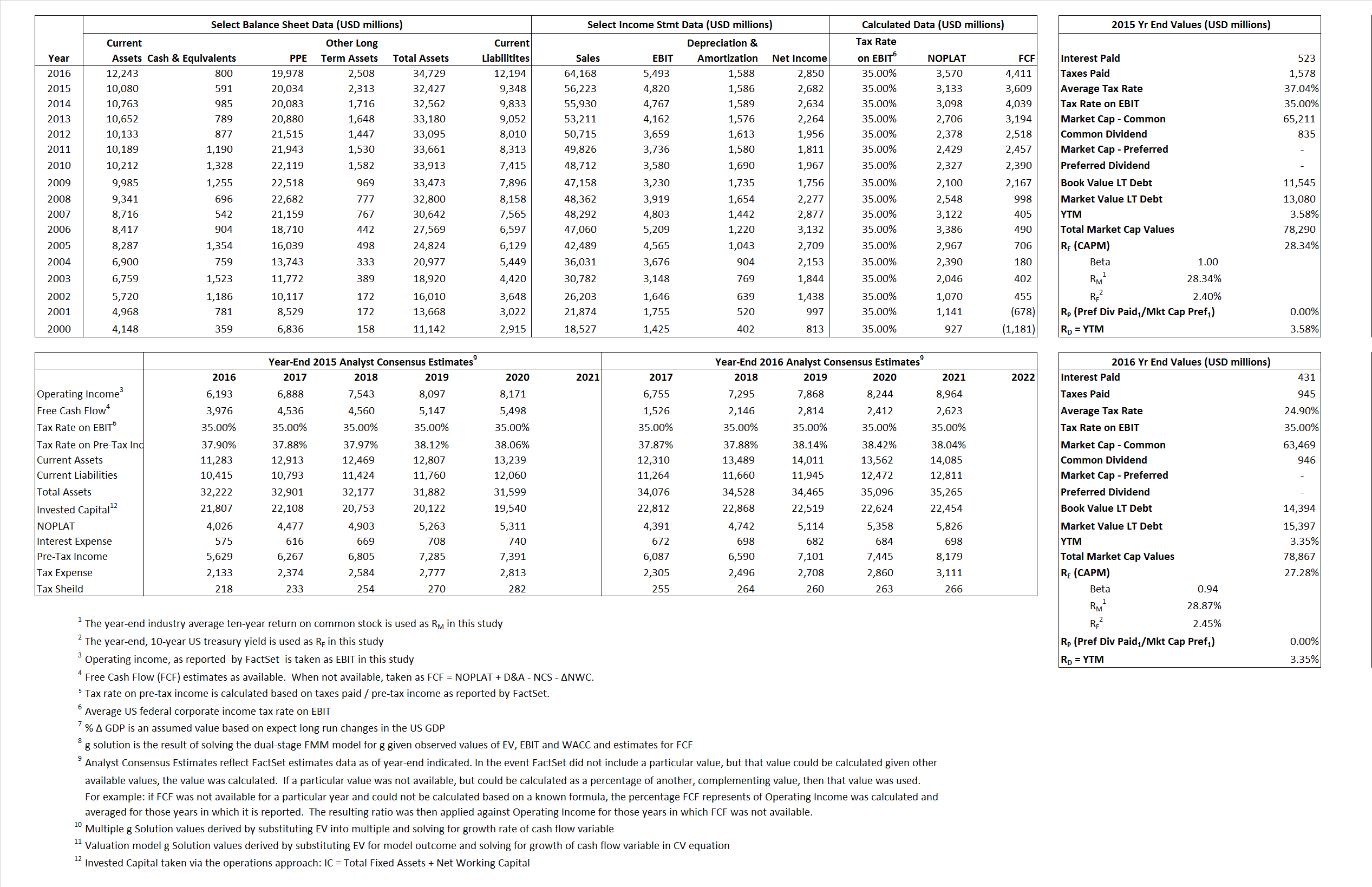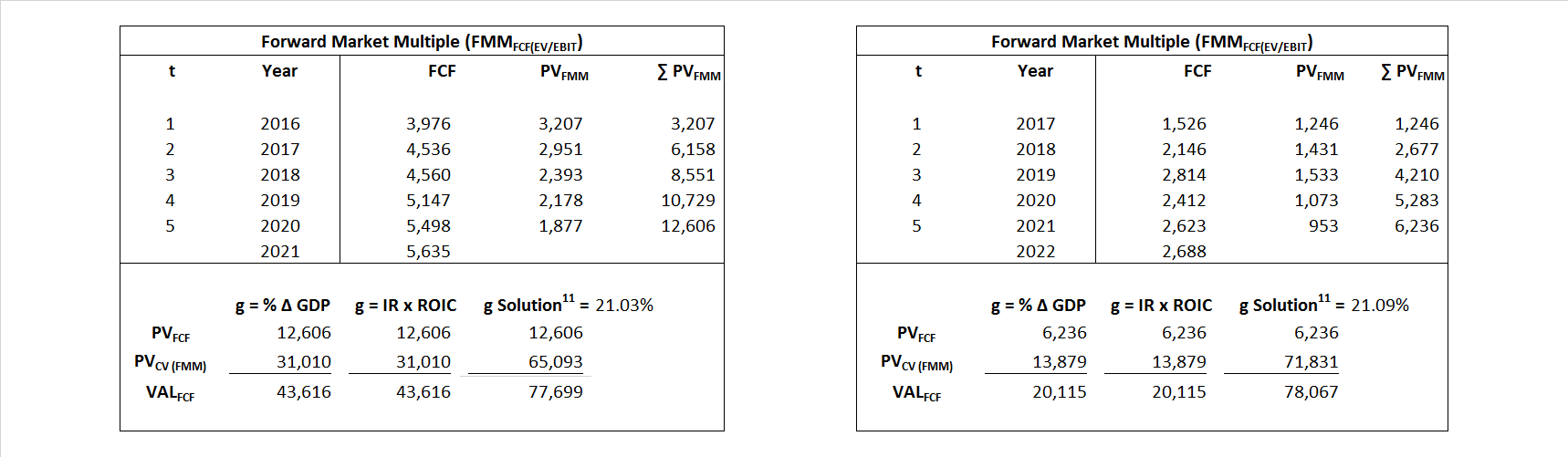# Lowe’s

## Analyst Listing

The following analysts provide coverage for the subject firm as of May 2016:

 Broker Analyst Analyst Email Oppenheimer Brian Nagel brian.nagel@opco.com Raymond James Budd Bugatch budd.bugatch@raymondjames.com Jefferies Daniel Binder dbinder@jefferies.com Consumer Edge Research David A. Schick dschick@consumeredgeresearch.com Longbow Research David S. MacGregor dmacgregor@longbowresearch.com Evercore ISI Greg Melich greg.melich@evercoreisi.com Nomura Research Jessica A. Schoen jessica.schoen@nomura.com Telsey Advisory Group Joseph Feldman jfeldman@telseygroup.com SunTrust Robinson Humphrey Keith Hughes keith.hughes@suntrust.com Deutsche Bank Research Mike Baker michael.baker@db.com Piper Jaffray Peter J. Keith peter.j.keith@pjc.com Atlantic Equities Sam Hudson s.hudson@atlantic-equities.com RBC Capital Markets Scot Ciccarelli scot.ciccarelli@rbccm.com Wolfe Research Scott Mushkin smushkin@wolferesearch.com Wedbush Securities Seth Basham seth.basham@wedbush.com BMO Capital Markets Wayne Hood wayne.hood@bmo.com

## Primary Input Data## Derived Input Data

### Equational Form

Net Operating Profit Less Adjusted Taxes NOPLAT  3,133   3,570$NOPLAT\, =\, EBIT\, x\, (1 \,-\, Avg \,\,Tax\,\, Rate\,\, on\,\, EBIT)$
Free Cash Flow FCF  3,609  4,411$FCF\,=NOPLAT\,+\,Non-Cash\,Expenses-\Delta NWC\,-\,NCS$
Tax Shield TS  194  107$TS\,=\,Interest\,\,Paid\,\,x\,\, Avg \,\,Tax\,\,Rate\,\, on\,\, Pre-Tax\,\, Income$
Invested Capital IC  23,079  22,535$IC\,=\,Fixed\,\,Operating\,\,Assets\,\,+\,\,Net\,\, Working\,\, Capital$
Return on Invested Capital ROIC 13.58% 15.84%$ROIC\,=\,\frac { NOPLAT }{ IC }$
Net Investment NetInv  1,936  1,044$NetInv\,=\,{ {IC}_{1}}-{{IC}_{0}}+Depreciation$
Investment Rate IR 61.80% 29.23%$IR\,=\,\frac {NetInv}{NOPLAT}$
Weighted Average Cost of Capital WACCMarket 23.98% 22.45%$WACC\,=\,\frac { E }{ V } { R }_{ E }\,+\,\frac { P }{ V } { R }_{ P }\,+\,\frac { D }{ V } { R }_{ D }\left( 1- Avg\,\, Tax\,\,Rate\,\,on\,\,Pre-Tax\,\,Income \right)$
WACCBook  7.31% 7.62%
Enterprise value EVMarket   77,699  78,067$EV\,=\,Market\,\,Cap\,\,Equity\,+\,\,Long\,\,Term\,\,Debt\,-\,Cash$
EVBook  75,956  77,063
EV/EBIT Multiple$\frac{EV_{Market}}{EBIT}$  16.12  14.21$EV/EBIT\,=\,\frac { EV}{ EBIT}$
Long-Run Growth g = IR x ROIC
8.39%   4.63% Long-run growth rates of the income variable  are used in the Continuing Value portion of the valuation models.
g = %$\Delta$ GDP    2.50%    2.50%

## Valuation Model Outcomes

The outcomes presented in this study are the result of original input data, derived data, and synthesized inputs and, depending on the equational form of any particular valuation model, may result in irrelevant or implausible results.  For example, in the event WACC < g, the value of this term, often found in the denominator of an equation’s continuation value term, will be expressly negative and may result in a negative overall valuation for the firm.  In the event of a WACC < g relation, the model form as applied to the subject firm offers an irrelevant outcome.

### Equational form

Key Value Driver (NOPLAT) KVD (NOPLAT)${ Value }_{ DCF/KVD }=\sum { \frac { NOPLAT_{ t } }{ { \left( 1+WACC \right) }^{ t } } +\frac { \frac { { NOPLAT }_{ 1 }\left( 1-\frac { g }{ ROIC } \right) }{ WACC-g } }{ { \left( 1+WACC \right) }^{ t } } }$Key Value Driver (FCF) KVD (FCF)${ Value }_{ DCF/KVD }=\sum { \frac { FCF_{ t } }{ { \left( 1+WACC \right) }^{ t } } +\frac { \frac { { NOPLAT }_{ 1 }\left( 1-\frac { g }{ ROIC } \right) }{ WACC-g } }{ { \left( 1+WACC \right) }^{ t } } }$Free Cash Flow FCF${ Value }_{ DCF/FCF }=\sum { \frac { FCF_{ t } }{ { \left( 1+WACC \right) }^{ t } } +\frac { \frac { { FCF }_{ 1 }}{ WACC-g } }{ { \left( 1+WACC \right) }^{ t } } }$Economic Profit ECON π${ Value }_{ { ECON\pi } }= I{ C }_{ 0 }+\sum { \frac { { IC }_{ t-1 }(ROI{ C }_{t}-WAC{C}_{t}) }{ { \left( 1+WACC \right) }^{ t } }+ \frac {\frac { I{C}_{0}\ x\ (ROI{C}_{1}\ -\ WAC{C}_{1}) }{ WACC-g } }{ { \left( 1+WACC \right) }^{ t } } }$Adjusted Present Value APV${ Value }_{ APV }=\sum { \frac { FCF_{ t } }{ { \left( 1+{ k }_{ u } \right) }^{ t } } +\frac { \frac { { FCF }_{ 1 }}{ { k }_{ u }-g } }{ { \left( 1+{ k }_{ u } \right) }^{ t } } } +\sum { \frac { { TS }_{ t } }{ { \left( 1+{ k }_{ tax } \right) }^{ t } } +\frac { \frac { { TS }_{ 1 }}{ { k }_{ tax }-g } }{ { \left( 1+{ k }_{ tax } \right) }^{ t } } }$Forward Market Multiple FMM${ Value }_{ DCF/FMM}=\sum { \frac { FCF_{ t } }{ { \left( 1+WACC \right) }^{ t } } +\frac { { EBIT }_{ 1 }\,{x}\,{FMM}}{ { \left( 1+WACC \right) }^{ t } } }{\,\,\,; \,\,FMM\,=\,\frac{{EV}_{t=0}}{{EBIT}_{t=0}}}$